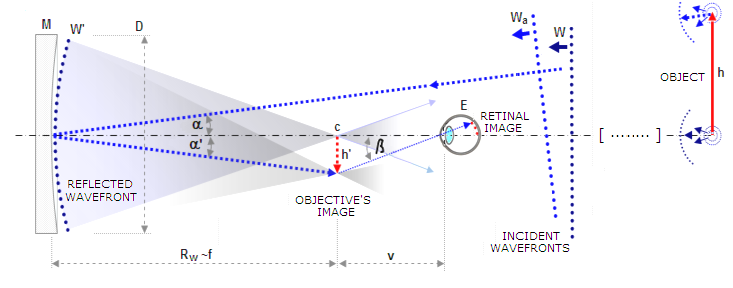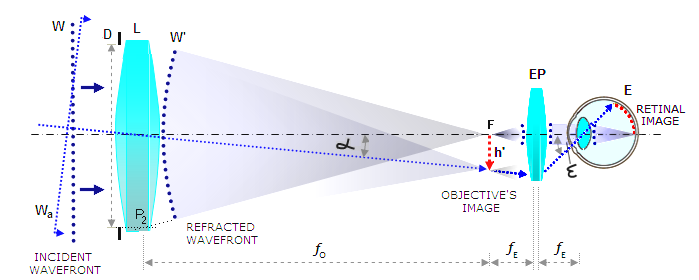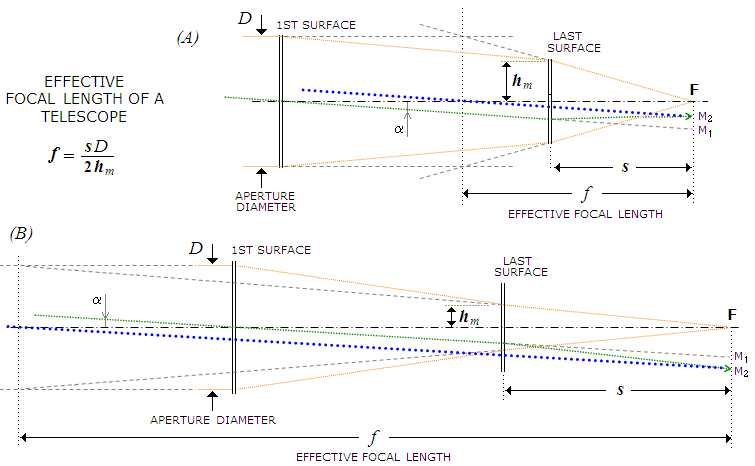telescopeѲptics.net          ▪▪▪▪                                             CONTENTS

1.3. Optical system of a telescopeOptical system of a telescope consists from:

(1) objective, which captures light from faraway objects and uses it to form their real image, and

(2) eyepiece, a sophisticated magnifying glass that enables the eye to greatly enlarge projection of this image onto the retina.

Telescope objective can be a single concave mirror; it can also consist from two or more mirrors, or lenses, or of mirror and lenses combined. It gathers light and forms images of distant objects. While the image it forms can be observed directly, the eye would only receive a small fraction of the light emerging from it (FIG. 6). It is the role of the ocular - or eyepiece - to make all the light from the image formed by the objective available to the eye and, by increasing apparent angles, add a significant magnification factor to the final image formed on the retina (FIG. 7).FIGURE 6: Image formed by a telescope mirror objective, as seen without the eyepiece (flat is omitted for simplicity). Wavefronts emitted from distant object of height h become practically flat over the aperture of a telescope, here a concave mirror M. The mirror changes the shape of the incoming flat wavefront section (W) into spherical (W') by delaying reflection of the points in phase belonging to the wavefront's inner area. Point of convergence (c) - or focal point - is at the center of curvature R of the wavefront. Thus, with the stop at mirror surface and exit pupil plane at mirror vertex, the mirror focal length equals the radius of the wavefront in the pupil.  The top point of distant object h, at an angle α from the optical axis, is imaged into (reversed) top of the image h' by off-axis wavefront Wa, originating at the object's top point. If observed directly, from the least distance of distinct vision v (approx. 25cm, or 10 inches) most of the light from the object image h' misses the eye pupil (more so for the points farther off-axis, with no light from the image's reversed top reaching the eye); also, magnification is limited to ƒ/v, ƒ being the objective's focal length. The apparent image angle β determines objective magnification as Mo=tanβ/tanα.FIGURE 7:
Image formed by a telescope lens objective, as seen through the eyepiece. Since light is slowed down in glass, the in-phase points of the incident axial wavefront W are retarded the most in the center of the lens objective L (for simplicity, both objective and eyepiece are shown as a single lens), and the least at its edges. Properly made lens objective will re-shape flat incident wavefront W into spherical (W') after exiting the lens. That is a goal for off-axis point wavefronts (Wa) as well, although some form of deviation due to tilt-created asymmetry is usually present. In terms of rays, change in direction of straight lines orthogonal to the wavefront (rays), resulting from its new shape, is called refraction. The lens objective focal length ƒO is the distance between its second principal point P2 and the focal point F. The eyepiece (EP), placed at a distance of its focal length ƒE from the object image h' formed by the objective, converts diverging spherical wavefront into flat, for which the eye has preference. It also increases the apparent incidence angle (α vs. ε), making the object imaged at the retina (E) appear larger by a factor O/ƒE. Use of an eyepiece allows for far more light from the object's image (all of it, if properly designed) to reach the eye, much higher magnifications, and much wider fields compared to observing image formed by the objective with eye alone.

The very basic element of a telescope is the diameter of its aperture. Given optical quality, it is the main determinant of telescope's capabilities with respect to light gathering and resolution, thus also of its limits in useful magnification. If well made, the eyepiece has no appreciable effect on the light gathering or inherent resolution of a telescope. Its main function is magnification of the real image formed by the objective. Consequently, the main optical parameters of a telescope relate to its objective. They are:

aperture diameter, hereafter denoted by D

focal length ƒ and

relative aperture D/ƒ=1/F, with F being the focal ratio

Thus, telescope consist from a single or or multi-element objective, and an eyepiece centered around the optical axis of the objective. The objective forms the focal point - a point of ray convergence on its optical axis (in terms of physical optics, the point of the highest generated wave energy, but the two generally coincide only in aberration-free system) - which determines the focal length of a telescope.

Telescope focal length is a distance from the objective to where it focuses collimated light. That is, when the light arrives from objects far enough that the wavefront entering the objective is practically flat, and the light rays are practically parallel. In complex objectives, the effective focal length is determined from the focus separation s from the last surface and the height of marginal ray hm on it, as ƒ=sD/2hm (FIG. 8). For closer objects, the focus forms farther away from the objective.FIGURE 8: Effective focal length of a telescope system with widely separated surfaces is obtained by extending the final converging axial cone (after the last surface) backward to the point of intersection with the height of the aperture. It can be either shorter (A) or longer (B) than the physical separation between the first surface and focus. The light convergence produced at the last surface bends the chief ray (green) to produce the final system magnification M2, proportional to its effective focal length, not the surface-to-focus separation.  A line connecting M2 with the effective (virtual) aperture center (dotted blue) forms an angle with the axis that is equal to the angle of incident light α. Linear size of the Airy disc is determined by the final angle of convergence (i.e. by the effective focal ratio), while its angular size (linear size divided with the effective focal length) remains that corresponding to the aperture diameter (i.e. 2.44λF/ƒ=2.44λ/D, in radians).

The definition of focal length implies that an optical surface, or system, will have a constant focal length only if it focuses all rays to a single point on the path of the central ray. No actual system does since, due to the presence of spherical aberration, the location of focus varies with the ray height in the telescope pupil. For a given aperture, the focal length is conventionally defined as that with respect to the focal point formed by its marginal (edge) rays. So, for a concave mirror of the vertex radius of curvature R, aperture radius d, surface sagitta z and conic constant K, the focal length is defined by:

2ƒ=R+(1-K)z-d2/[R-(1+K)z)]

For spherical mirror, K=0 and 2ƒ=R+z-d2/(R-z) which, knowing that the last term closely approximates 2z, implies ƒ<R/2, with the differential between ƒ for d0 and for d»0 defining the separation between two focus location, i.e. longitudinal spherical aberration. The change in focal length for d»0 (marginal rays) vs. d0 (paraxial) is opposite in sign to R, implying marginal focus closer to mirror than paraxial (under-correction).

For a paraboloid, K=-1 and 2ƒ=R+2z-d2/R which, knowing that z=d2/2R for parabola, yields 2ƒ=R and ƒ= R/2, with zero spherical aberration.

For K=-2 (hyperboloid), 2ƒ=R+3z-d2/(R+z), with the same magnitude of longitudinal aberration as sphere, only with marginal rays focusing longer than paraxial (over-correction).

Similarly, with object at infinity, any lens with spherical surfaces generates spherical aberration (positive lens under-correction, negative over-correction).

In determining the basic image properties - its location with respect to imaging element, and size (first-order optics) - system aberrations are not a significant factor and can be neglected. The simplified calculation following light passing through the central, practically aberration-free portion of the optical surface/element is called paraxial, or Gaussian approximation.